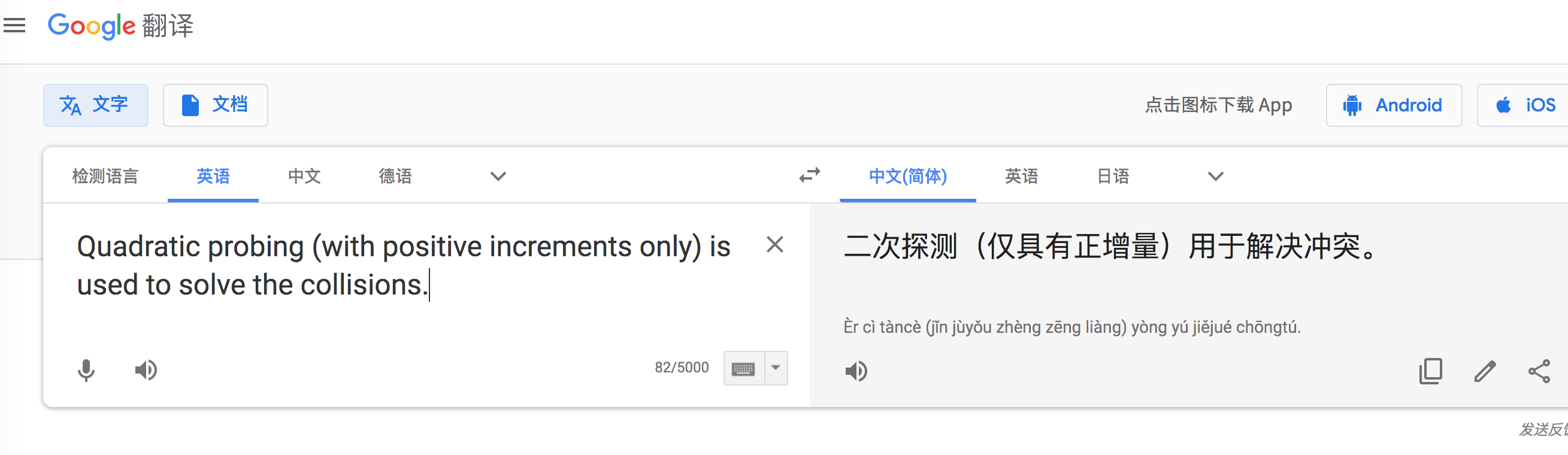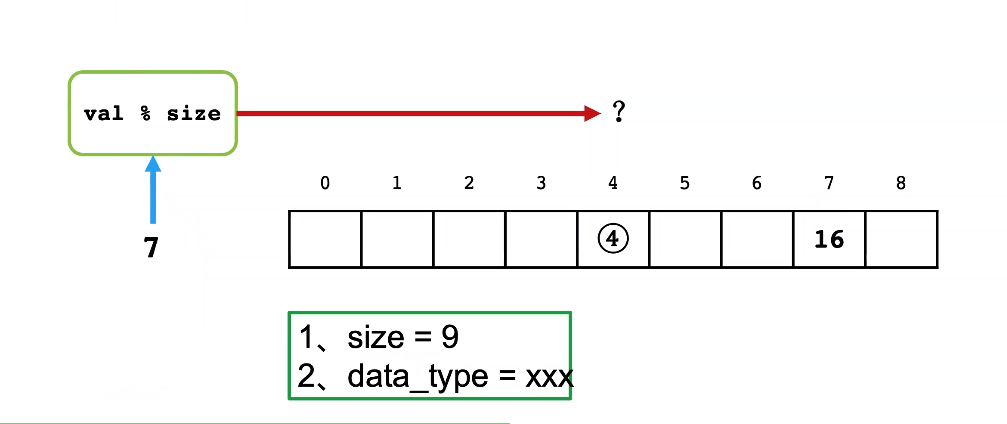• 15-C. DS哈希查找—二次探测再散列
2021-12-22 13:00:18

实验15-哈希查找与排序-
题目描述
定义哈希函数为H(key) = key%11。输入表长（大于、等于11），输入关键字集合，用二次探测再散列构建哈希表，并查找给定关键字。

输入
测试次数t

每组测试数据格式如下：

哈希表长m、关键字个数n

n个关键字

查找次数k

k个待查关键字

输出
对每组测试数据，输出以下信息：

构造的哈希表信息，数组中没有关键字的位置输出NULL

对k个待查关键字，分别输出：

0或1（0—不成功，1—成功）、比较次数、查找成功的位置（从1开始）

输入样例
1
12 10
22 19 21 8 9 30 33 4 41 13
4
22
15
30
41

输出样例
22 9 13 NULL 4 41 NULL 30 19 8 21 33
1 1 1
0 3
1 3 8
1 6 6

#include<iostream>
using namespace std;

class Hash
{
int *T;//哈希表
int m;//表长
int n;//关键字数
public:
Hash(int v1,int v2){m=v1;T=new int[m];n=v2;}
void createhash()
{
int i,e;
for(i=0;i<m;i++)
T[i]=-1;

for(i=0;i<n;i++)//输入n个关键字
{
int sum=0,flag=0;//sum是乘数
cin>>e;
if(T[e%11]==-1)
{
T[e%11]=e;
}
else
{
while(1)
{
int pos;
flag++;
if(flag>=2&&flag%2==0)
sum++;//每两次乘数++

if(flag%2==1)//flag是奇数的时候-
pos=(e%11-sum*sum)%m;
else
pos=(e%11+sum*sum)%m;
while(pos<0)
pos+=m;//不用在pos>m时候-=m，因为已经%m过

if(T[pos]==-1)
{
T[pos]=e;
break;
}

}
}
}
for(i=0;i<m;i++)
{
if(T[i]==-1)
cout<<"NULL";
else
cout<<T[i];

if(i==m-1)
cout<<endl;
else
cout<<" ";
}
}
void search(int e)
{
//需要输出：成功与否，比较次数，查找成功的位置
//成功1失败0，比较次数flag，查找成功的位置(e%11+sum*sum*c)%m+1(位置从1开始！)
int sum=0,flag=0,c;
while(1)
{
flag++;
if(flag>=2&&flag%2==0)
sum++;

if(flag%2==0)
c=1;
else
c=-1;

int p=(e%11+c*sum*sum)%m;
if(T[p]==e)
{
cout<<"1 "<<flag<<" "<<p+1<<endl;
break;
}
else if(flag==n||T[p]==-1)
{
cout<<"0 "<<flag<<endl;
break;
}
}
}
};

int main()
{
int t,n,m,k;
cin>>t;
while(t--)
{
cin>>m>>n;//表长m 关键字n
Hash H(m,n);
H.createhash();

cin>>k;//查找次数k
while(k--)
{
int e;
cin>>e;
H.search(e);
}
}
return 0;
}c语言 散列表
更多相关内容
• 输入表长（大于、等于11），输入关键字集合，用二次探测再散列构建哈希表，并查找给定关键字。探测时如遇到已存在于哈希表的相同数字，则新数字不存入。 输入 测试次数t 每组测试数据格式如下： 哈希表长m、关键字个...

## 题目描述

定义哈希函数为H(key) = key%11。输入表长（大于、等于11），输入关键字集合，用二次探测再散列构建哈希表，并查找给定关键字。探测时如遇到已存在于哈希表的相同数字，则新数字不存入。

测试次数t
每组测试数据格式如下：
哈希表长m、关键字个数n
n个关键字
查找次数k
k个待查关键字

## 输出

对每组测试数据，输出以下信息：
构造的哈希表信息，数组中没有关键字的位置输出NULL
对k个待查关键字，分别输出：
0或1（0—不成功，1—成功）、比较次数、查找成功的位置（从1开始）

## 样例输入1

1
12 10
22 19 21 8 9 30 33 4 41 13
4
22
15
30
41

## 样例输出1

22 9 13 NULL 4 41 NULL 30 19 8 21 33
1 1 1
0 3
1 3 8
1 6 6

## 样例输入2

1
79 79
8 5 26 26 12 12 16 28 13 17 26 16 7 29 27 23 3 29 23 28 21 9 18 19 11 27 0 6 5 10 15 5 8 3 1 20 15 17 19 28 26 7 6 3 7 25 18 2 16 11 22 7 13 10 19 24 7 19 22 4 22 7 9 22 2 2 12 17 12 23 7 8 23 13 12 0 0 22 24
26
17
4
16
24
17
18
5
3
25
17
25
0
9
25
1
1
27
4
5
7
16
29
6
24
14
12

## 样例输出2

23 12 13 29 26 5 16 28 8 27 17 7 19 9 21 6 18 NULL 20 10 4 NULL NULL NULL NULL NULL NULL NULL NULL NULL NULL NULL NULL NULL NULL NULL NULL NULL NULL NULL NULL NULL NULL NULL NULL NULL NULL NULL NULL NULL NULL NULL NULL NULL NULL NULL NULL NULL NULL NULL NULL NULL NULL 22 NULL NULL NULL NULL NULL NULL 0 NULL 24 25 15 11 1 2 3
1 4 11
1 8 21
1 2 7
1 7 73
1 4 11
1 6 17
1 1 6
1 5 79
1 7 74
1 4 11
1 7 74
1 7 71
1 4 14
1 7 74
1 5 77
1 5 77
1 4 10
1 8 21
1 1 6
1 4 12
1 2 7
1 5 4
1 6 16
1 7 73
0 9
1 1 2

## 代码示例

注意下面代码的标注的地方**

#include <iostream>
using namespace std;

#define INITIALIZE -1

class HashTable
{
private:
int size;			// 表长
int* hashTable;		// 哈希表，用于存值
public:
HashTable();
~HashTable();
int hash(int num);
void outPut();
void searchValue(int t);
};

HashTable::HashTable()
{
int n;				// 关键字个数
cin >> size >> n;
hashTable = new int[size];
// 初始化哈希表
for (int i = 0; i < size; i++)
{
hashTable[i] = INITIALIZE;	// 初始化哈希表，表示为空
}
// 构建哈希表
int val;
int key;
while (n--)
{
cin >> val;
key = hash(val);		// 据哈希函数求键值
int index = key % size;
if (hashTable[index] == INITIALIZE || hashTable[index] == val)
{
hashTable[index] = val;
}
else
{
int oper = 0;
for (int i = 1; ; i++)	// 二次探测
{
oper = i * i;
while (oper > size)		//**注意；这里如果没有这样操作，在输入案例很多的情况下，会导致后面数组访问越界
{
oper -= size;
}
if (hashTable[(index + oper) % size] == INITIALIZE || hashTable[(index + oper) % size] == val)	// **注意这里需要对重复值进行判断，即当表已经出现这个值的时候，不要再往下找了
{
hashTable[(index + oper) % size] = val;
break;
}
else
{
if ((index - oper) >= 0)
{
if (hashTable[index - oper] == INITIALIZE || hashTable[index - oper] == val)
{
hashTable[index - oper] = val;
break;
}
}
else
{
if (hashTable[size + (index - oper)] == INITIALIZE || hashTable[size + (index - oper)] == val)//
{
hashTable[size + (index - oper)] = val;
break;
}
}
}
}
}
}
}

HashTable::~HashTable()
{
delete[] hashTable;
}

// 哈希函数
int HashTable::hash(int num)
{
return num % 11;
}

void HashTable::outPut()
{
for (int i = 0; i < size; i++)
{
if (hashTable[i] != INITIALIZE)
{
cout << hashTable[i];
}
else
{
cout << "NULL";
}
// 用这种方式输出空格，可以避免输出最后一个值的时候多输出额外空格的问题发生
if (i != size - 1)
{
cout << ' ';
}
}
cout << endl;
}

void HashTable::searchValue(int t)
{
int searchTime;	// 比较次数
int val;		// 需要被查找的值
int key;		// 值对应的键
int flag;		// 标记是否成功查找：0 或 1（0—不成功，1—成功）
while (t--)
{
searchTime = 0;
flag = 0;
cin >> val;
key = hash(val);	// 据哈希函数求键值
int index = key;
int symbol = 1;		// 1 代表 “正号”，0代表“负号”
// 查找关键代码
int oper = 0;
for (int i = 1;;)		// 反复二次探测查找
{
searchTime++;
if (hashTable[index] == val)				// 查找成功
{
flag = 1;
cout << 1 << ' ' << searchTime << ' ' << (index)+1 << endl;	// 查找成功的位置：从1开始，需要index + 1
break;
}
else if (hashTable[index] == INITIALIZE)
{
break;
}
oper = i * i;
while (oper > size)
{
oper -= size;
}
if (symbol)
{
symbol = 0;			// 下次变成“负号”
index = (key + oper) % size;
}
else
{
symbol = 1;
index = key - oper;
if (index < 0)
{
index += size;
}
i++;						// 注意i增加放置的位置
}
}
if (flag == 0)
{
// 不能将下面语句该输出放在①处；原因：如果hashTable是慢的，且没有需要查找的元素，
// 此时将输出放置①处会导致没有结果输出，所以将输出独立出来。
cout << 0 << ' ' << searchTime << endl;
}
}
}

int main()
{
int t;
cin >> t;
while (t--)
{
HashTable table;
table.outPut();
int times;
cin >> times;
table.searchValue(times);
}

return 0;
}

展开全文散列表 算法
•代码
• 1078 Hashing (25 分) 题目传送门：1078 Hashing (25 分) ...在哈希时如果冲突，则二次探测再散列。刚开始没注意这一句:Quadratic probing (with positive increments only) is used to solve the...

# 1078 Hashing (25 分)

题目传送门：1078 Hashing (25 分)

## 一、题目大意

将给定长度为n的数组放到长度为M的哈希表中，如果M不是素数，则将M往后扩大到M后面的第一个素数。
在哈希时如果冲突，则二次探测再散列。刚开始没注意这一句:Quadratic probing (with positive increments only) is used to solve the collisions.，以至于最后一组数据一直过不了。## 二、解题思路

将M扩增到大于等于的M的最小素数不难，难点是不知道什么是哈希表二次探测再散列，我百度后才知道，其实就是当将key往哈希表中存的时候，当发现key%M这一位已经存放了别的数了，那再放key就会产生冲突，那么我们该怎么解决冲突呢，就是二次探测，就是依次看(key±i^2)%M是否存放数，i是从1到M-1，如果遇到一个未存放数的哈希位置，我们就把key放到放到(key±i^2)%M这个位置上即可。

## 三、AC代码

#include<bits/stdc++.h>
using namespace std;
vector<int>P{2};
void init(int n){//滚动数组产生素数表
for(int i = P.back()+1; i <= n; i+=2){
bool f = true;
for(auto j: P){
if(i % j == 0){
f = false;
break;
}
}
if(f){
P.push_back(i);
}
}
}
int main(){
int M, N;
cin >> M >> N;
if(M == 1)M++;
init(M);
if(P.back() != M){
for(int i = M+1; ; i++){
bool f = true;
for(auto j: P){
if(i % j == 0){
f = false;
break;
}
}
if(f){
P.push_back(i);
break;
}
}
M = P.back();
}
vector<int>v(N), hash(M);
for(int i = 0; i < N; i++){
int x;
cin >> x;
if(hash[x%M] == 0){// 原位存入
hash[x%M] = x;
v[i] = x % M;
}else{// 冲突，二次探测
bool f = true;
for(int j = 1; j < M; j++){
int y = x + j*j;
if(hash[y%M] == 0){
hash[y%M] = x;
v[i] = y%M;
f = false;
break;
}
}
if(f)// 二次探测后依旧冲突无法存放
v[i] = -1;
}
}
for(int i = 0; i < N; i++){
if(i){
if(v[i]==-1){
cout << " -";
}else{
cout << " " << v[i];
}
}else{
if(v[i]==-1){
cout << "-";
}else{
cout << v[i];
}
}
}
cout << endl;
}

展开全文• 输入表长（大于、等于11），输入关键字集合，用二次探测再散列构建哈希表，并查找给定关键字。 输入 测试次数t 每组测试数据格式如下： 哈希表长m、关键字个数n n个关键字 查找次数k k个待查关键字 输出 对每组测试...

题目描述

定义哈希函数为H(key) = key%11。输入表长（大于、等于11），输入关键字集合，用二次探测再散列构建哈希表，并查找给定关键字。

输入

测试次数t

每组测试数据格式如下：

哈希表长m、关键字个数n

n个关键字

查找次数k

k个待查关键字

输出

对每组测试数据，输出以下信息：

构造的哈希表信息，数组中没有关键字的位置输出NULL

对k个待查关键字，分别输出：

0或1（0—不成功，1—成功）、比较次数、查找成功的位置（从1开始）

样例输入

1

12 10

22 19 21 8 9 30 33 4 41 13

4

22

15

30

41

样例输出

22 9 13 NULL 4 41 NULL 30 19 8 21 33

1 1 1

0 3

1 3 8

1 6 6二次探测再散列
如图 的位置已经存放了16， 那么7 存放的位置 就在7+12, 7-12,7+22,7-22,7+32,7-32找到NULL为止
因为7+12 =  = NULL， 所以7 放在位置

#include <iostream>
using namespace std;

class HashTable {
private:
int *data;
int size;
public:
HashTable(int n) {
size = n;
data = new int[size];
for (int i = 0; i < size; i++) {
data[i] = -1;
}
}
int Hash(int n) {
return n%11;
}
void insert(int n, int hash) {
hash %= size;
if(data[hash] == -1) {
data[hash] = n;
} else {
int i = 1;
while(1) {
if(data[(hash + i*i) % size] == -1) {
data[(hash + i*i) % size] = n;
break;
} else {
if((hash - i*i) >= 0){
if(data[(hash - i*i)] == -1){
data[(hash - i*i)] = n;
break;
}
}else{
if(data[size + (hash - i*i)] == -1){
data[size + (hash - i*i)] = n;
break;
}
}
}
i++;
}
}
}
void search(int find) {
int cnt = 0, index = 0;
int hash = Hash(find);
int i = 1;
int flag = 1,sum = hash;
while(1) {  //反复横挑的查找 + 1^2 , - 1^2 ......
cnt++;
if(data[sum] == find) {
cout<<"1 "<<cnt<<" "<<sum + 1<<"\n";
return ;
}
if(data[sum] == -1) {
cout<<"0 "<<cnt<<"\n";
return ;
}
if(flag){ //判断是+还是-
flag = 0;
sum = hash + i * i; //横跳的位置
sum %= size;
}else{
flag = 1;
sum = hash - i * i; //横跳的位置
if(sum < 0){
sum = size + sum;
}
i++;
}

}
}
void print() {
for(int i = 0; i < size; i++) {
if(data[i] > 0) {
cout<<data[i]<<" ";
} else {
cout<<"NULL ";
}
}
cout<<endl;
}
~HashTable() {
delete[] data;
}
};

int main() {
int t;
cin>>t;
while(t--) {
int size,n;
cin>>size>>n;
HashTable h(size);
while(n--) {
int data;
cin>>data;
h.insert(data,h.Hash(data));
}
h.print();
int k;
cin>>k;
while(k--) {
int find;
cin>>find;
h.search(find);
}
}
return 0;
}

展开全文数据结构 算法 hash
• 　（2）从键盘读入待查找的权重数值，以除留余数法为哈希函数，二次探测再散列法解决冲突建立哈希表，基于哈希算法从数组中查找相应的记录，计算相应的查找时间，并在屏幕上输出显示。(提示:当前计算机时间 函数 C\...
• 问题描述从空表开始，将输入元素按照输入顺序逐个插入一个哈希表，以生成哈希表...=m)，用二次探测再散列法处理冲突，即探测序列为Hi=(Hash(key)+di) mod m，其中增量序列为di = 12, -12, 22, -22,32,-32…, k2, -k2...
• 最近复习了下数据结构中的哈希表，发现在计算等概率情况下查找不成功的平均查找长度时比较迷茫，不知道到底是怎么计算出来的。现在通过查阅资料终于知道如何计算了，所以记录下来以供以后查阅。  下面看下2010年...
• 线性探测再散列时 以 存储空间的长度来取余 查找时比较次数，如在 {12}中查找12，12跟12也要进行一比较。 Question1: 将关键字序列（7、8、30、11、18、9、14）散列存储到散列表中。散列表的存储空间是一个下标从0...
• 11 个关键字，16，74，60，43，54，90，46，31，29，88，77，构造哈希表    如图，例如 16%13=3，将16放入3号位置，29%13 = 3，将29放入3号位置，而此时3号位已经有元素。 就顺着表往后放，直到6...
• 散列表，也称为哈希表。根据设定的哈希函数H（key）和处理冲突的方法将一组... 处理冲突的方法：1）开放定址法（线性探测再散列二次探测再散列，伪随机探测再散列） 2）哈希法 3）链地址法 4）建立一 公共溢出区
• 散列表的存储空间是一个下标从0开始的一维数组，散列函数为： H(key) = (keyx3) MOD 7，处理冲突采用线性探测再散列法，要求装填（载）因子为0.7。 (1) 请画出所构造的散列表。 (2) 分别计算等概率情况下查找成功和...算法
• 前面的文章分析了开地址法的其中一种：线性探测再散列，这篇文章来讲开地址法的第二种：二次探测再散列(二)、二次探测再散列为改善“堆积”问题，减少为完成搜索所需的平均探查次数，可使用二次探测法。通过某一个...
• 问的是至少需要多少次探测，即我们假设在上一次探测的基础上，每进行一次二次探测都能直接找到对应的位置。 第一个：直接找到位置，入坑，1次； 第二个：和第一个同hash，找到的位置被第一个给占了，通过二次探测...java
• ## 二次探测散列法

千次阅读 2020-12-28 21:36:29
• 题目来源：2010-408_计算机学科专业基础综合 链接：...将关键字序列(7 . 8 . 30 . 11 . 18 . 9 . 14)散列存储到散列表中，散列表的存储空间是一个下标从0开始的一维数组 。 散...
• 它通过一个关键值的函数将所需的数据直接映射到表中的位置来访问数据，这个映射函数叫散列函数（哈希函数），存放记录的数组叫散列表（哈希表）。比如： 给定一字符串“abckhdgubhkacdggk”找出第一只出现一的...hashtable 数据结构
• 大侠们救命----关于二次探测再散列的C语言实现(2012-06-05 01:08:07)标签：二c语言杂谈大侠们救命----关于二次探测再散列的C语言实现针对开放地址哈希表结构，采用线性探测再散列处理冲突过程中会发生的两个第一个......